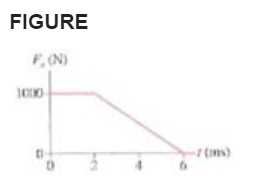# Problem: What impulse does the force shown in FIGURE exert on a 250 g particle?

###### FREE Expert Solution

Impulse is equal to the area under the curve.

Based on the approach, we do not need to know the mass of the object to calculate the impulse.

93% (321 ratings)###### Problem Details

What impulse does the force shown in FIGURE exert on a 250 g particle?